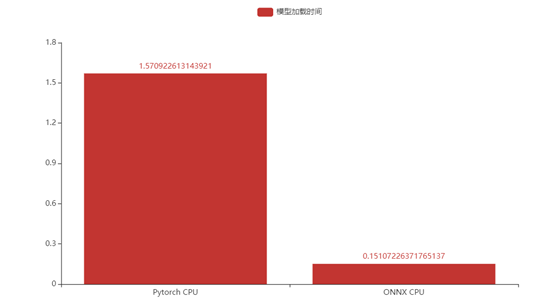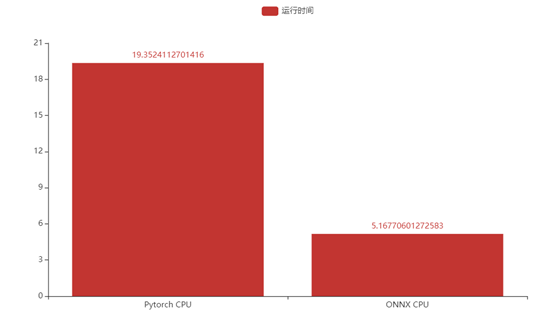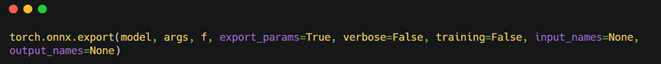Ericam_blog

11## 一. 简介

ONNX （Open Neural Network Exchange）- 开放神经网络交换格式，作为框架共用的一种模型交换格式，使用 protobuf 二进制格式来序列化模型，可以提供更好的传输性能我们可能会在某一任务中将 Pytorch 或者 TensorFlow 模型转化为 ONNX 模型(ONNX 模型一般用于中间部署阶段)，然后再拿转化后的 ONNX模型进而转化为我们使用不同框架部署需要的类型，ONNX 相当于一个翻译的作用。

## 二. ONNX结构分析

ONNX将每一个网络的每一层或者说是每一个算子当作节点Node，再由这些Node去构建一个Graph，相当于是一个网络。最后将Graph和这个onnx模型的其他信息结合在一起，生成一个model，也就是最终的.onnx的模型。
构建一个简单的onnx模型，实质上，只要构建好每一个node，然后将它们和输入输出超参数一起塞到graph，最后转成model就可以了。

## 三. ONNX 工作原理

在计算方面，虽然更高级的表达不同，但不同框架产生的最终结果都是非常接近。因此实时跟踪某一个神经网络是如何在这些框架上生成的，接着使用这些信息创建一个通用的计算图，即符合ONNX标准的计算图。

ONNX为可扩展的计算图模型、内部运算器（Operator）以及标准数据类型提供了定义。在初始阶段，每个计算数据流图以节点列表的形式组织起来，构成一个非循环的图。节点有一个或多个的输入与输出。每个节点都是对一个运算器的调用。图还会包含协助记录其目的、作者等信息的元数据。运算器在图的外部实现，但那些内置的运算器可移植到不同的框架上，每个支持ONNX的框架将在匹配的数据类型上提供这些运算器的实现。

## 四. 如何查看onnx网络结构和参数？## 五. 推理速度对比Pytorch下完成整个测试集推理时间需要19.35s，而ONNX则需要5.16s，推理速度大约提升2.75倍。## 六. 使用方法

### 1.环境配置

pip install onnx
pip install onnxruntime


### 2.将pytorch模型导出到ONNX格式• model(torch.nn.Module)-要被导出的模型
• args(参数的集合)-模型的输入，例如，这种model(*args)方式是对模型的有效调用。任何非Variable参数都将硬编码到导出的模型中；任何Variable参数都将成为导出的模型的输入，并按照他们在args中出现的顺序输入。如果args是一个Variable，这等价于用包含这个Variable的1-ary元组调用它。(注意:现在不支持向模型传递关键字参数。)
• f - 一个类文件的对象(必须实现文件描述符的返回)或一个包含文件名字符串。一个二进制Protobuf将会写入这个文件中。
• export_params(bool,default True)-如果指定，所有参数都会被导出。如果你只想导出一个未训练的模型，就将此参数设置为False。在这种情况下，导出的模型将首先把所有parameters作为参arguments，顺序由model.state_dict().values()指定。
• verbose(bool,default False)-如果指定，将会输出被导出的轨迹的调试描述。
• training(bool,default False)-导出训练模型下的模型。目前，ONNX只面向推断模型的导出，所以一般不需要将该项设置为True。
• input_names(list of strings, default empty list)-按顺序分配名称到图中的输入节点。
• output_names(list of strings, default empty list)-按顺序分配名称到图中的输出节点。
batch_size = 1  #批处理大小
input_shape = (1,28,28)   #输入数据
# set the model to inference mode
model.eval().cuda()
x = torch.randn(batch_size,*input_shape).cuda()		# 生成张量
export_onnx_file = "./mnist.onnx"					# 目的ONNX文件名
torch.onnx.export(model,
x,
export_onnx_file,
opset_version=10,
do_constant_folding=True,	# 是否执行常量折叠优化
input_names=["input"],		# 输入名
output_names=["output"],	# 输出名
dynamic_axes={"input":{0:"batch_size"},		# 批处理变量
"output":{0:"batch_size"}})


### 3.引入ONNX模型

class ONNXModel():
def __init__(self, onnx_path):
"""
:param onnx_path:
"""
self.onnx_session = onnxruntime.InferenceSession(onnx_path)
self.input_name = self.get_input_name(self.onnx_session)
self.output_name = self.get_output_name(self.onnx_session)
print("input_name:{}".format(self.input_name))
print("output_name:{}".format(self.output_name))

def get_output_name(self, onnx_session):
"""
output_name = onnx_session.get_outputs().name
:param onnx_session:
:return:
"""
output_name = []
for node in onnx_session.get_outputs():
output_name.append(node.name)
return output_name

def get_input_name(self, onnx_session):
"""
input_name = onnx_session.get_inputs().name
:param onnx_session:
:return:
"""
input_name = []
for node in onnx_session.get_inputs():
input_name.append(node.name)
return input_name

def get_input_feed(self, input_name, image_numpy):
"""
input_feed={self.input_name: image_numpy}
:param input_name:
:param image_numpy:
:return:
"""
input_feed = {}
for name in input_name:
input_feed[name] = image_numpy
return input_feed

def forward(self, image_numpy):
'''
# image_numpy = image.transpose(2, 0, 1)
# image_numpy = image_numpy[np.newaxis, :]
# onnx_session.run([output_name], {input_name: x})
# :param image_numpy:
# :return:
'''
# 输入数据的类型必须与模型一致,以下三种写法都是可以的
# scores, boxes = self.onnx_session.run(None, {self.input_name: image_numpy})
# scores, boxes = self.onnx_session.run(self.output_name, input_feed={self.input_name: iimage_numpy})
input_feed = self.get_input_feed(self.input_name, image_numpy)
scores = self.onnx_session.run(self.output_name, input_feed=input_feed)
return scores

def to_numpy(tensor):
#print(tensor.device)
return tensor.detach().cpu().numpy() if tensor.requires_grad else tensor.cpu().numpy()


### 4.推理计算

r_model_path = "./mnist.onnx"
time_start1 = time.time()
rnet1 = ONNXModel(r_model_path)
time_end2 = time.time()
print('load model cost', time_end2 - time_start1)
# 测时间
test_correct = 0
time_start = time.time()
img,lable = Variable(img),Variable(label)
out = rnet1.forward(to_numpy(img))
pred = np.argmax(out)
correct = 0
if label.item()==pred:
correct+=1
test_correct += correct
time_end=time.time()
print("[{}/{}]".format(test_correct,len(test_datasets)))
print('infer cost',time_end-time_start)


## 七. 其他关于ONNX的基本操作

### 1. 获取onnx模型的输出层

import onnx
# 加载模型
# 检查模型格式是否完整及正确
onnx.checker.check_model(model)
# 获取输出层，包含层名称、维度信息
output = self.model.graph.output
print(output)



### 2.获取中节点输出数据

import onnx
from onnx import helper
# 加载模型
# 创建中间节点：层名称、数据类型、维度信息
prob_info = helper.make_tensor_value_info('layer1',onnx.TensorProto.FLOAT, [1, 3, 320, 280])
# 将构建完成的中间节点插入到模型中
model.graph.output.insert(0, prob_info)
# 保存新的模型
onnx.save(model, 'onnx_model_new.onnx')

# 扩展：
# 删除指定的节点方法： item为需要删除的节点
# model.graph.output.remove(item)



## 八.参考

• https://github.com/onnx/onnx

• https://github.com/Azure/MachineLearningNotebooks/blob/master/how-to-use-azureml/deployment/onnx/onnx-inference-mnist-deploy.ipynb

• https://www.onnxruntime.ai/python/auto_examples/index.html

• https://netron.app/

• https://www.cnblogs.com/Ryan0v0/p/12333487.html